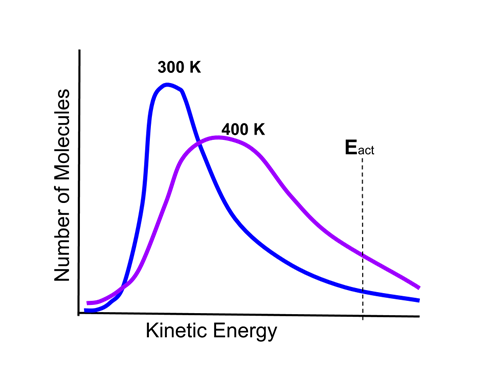# Enduring Understanding 4.B.2: Boltzmann & Arrhenius

• To overcome the energy barrier of a reaction, a particle must have sufficient energy.
• Reaction mixtures contain a very large number of particles with differing amounts of energy.
• The distribution of energies of particles can be described by the Maxwell-Boltzmann thermal distribution of particle energies. This is illustrated in the following graphic:

•• There are two curves on the graph, one representing a sample of reactant gas molecules at a temperature of 300 K, and the other the same sample at a temperature of 400 K.
• There is also an activation energy threshold of a reaction, Eact, indicated.
• Molecules with energy above Eact will have sufficient energy to undergo a reaction. As the graph shows, there are more molecules above Eact at 400 K than at 300 K, therefore the reaction will occur more rapidly at the higher temperature

• The Arrhenius equation is a mathematical function that gives the rate of a reaction, as a function of the activation energy Ea and the temperature T.
• K = Ae-Ea/RT

• A and R are constants.

 Related Links: Chemistry Chemistry Quizzes AP Chemistry Notes Reaction Mechanism AP Chemistry Quizzes Atoms, Molecules, and Chemical Analysis Quiz Electronic Structure of Atoms Quiz Periodicity, Atomic & Ionic Radii, Atomic Models Quiz Conservation of Matter and Balanced Equations Quiz Gases and Solutions Quiz Intermolecular Forces Quiz Molecular Geometry and VSEPR Quiz Bonding and Solid State Properties Quiz Balanced and Ionic Equations Quiz Chemical Reactions Quiz Redox Reactions and Electrochemistry Quiz Chemical Kinetics and Rates Quiz Reaction Rates and Collisions Quiz Multi-Step Reaction Rates and Catalysis Quiz Energy and Heat Quiz Conservation of Energy and Calorimetry Quiz Bond Energies Quiz Entropy and Gibbs Free Energy Quiz Chemical Equilibrium Quiz Solubility and Acid-Base Equilibrium Quiz

To link to this Boltzmann & Arrhenius page, copy the following code to your site: